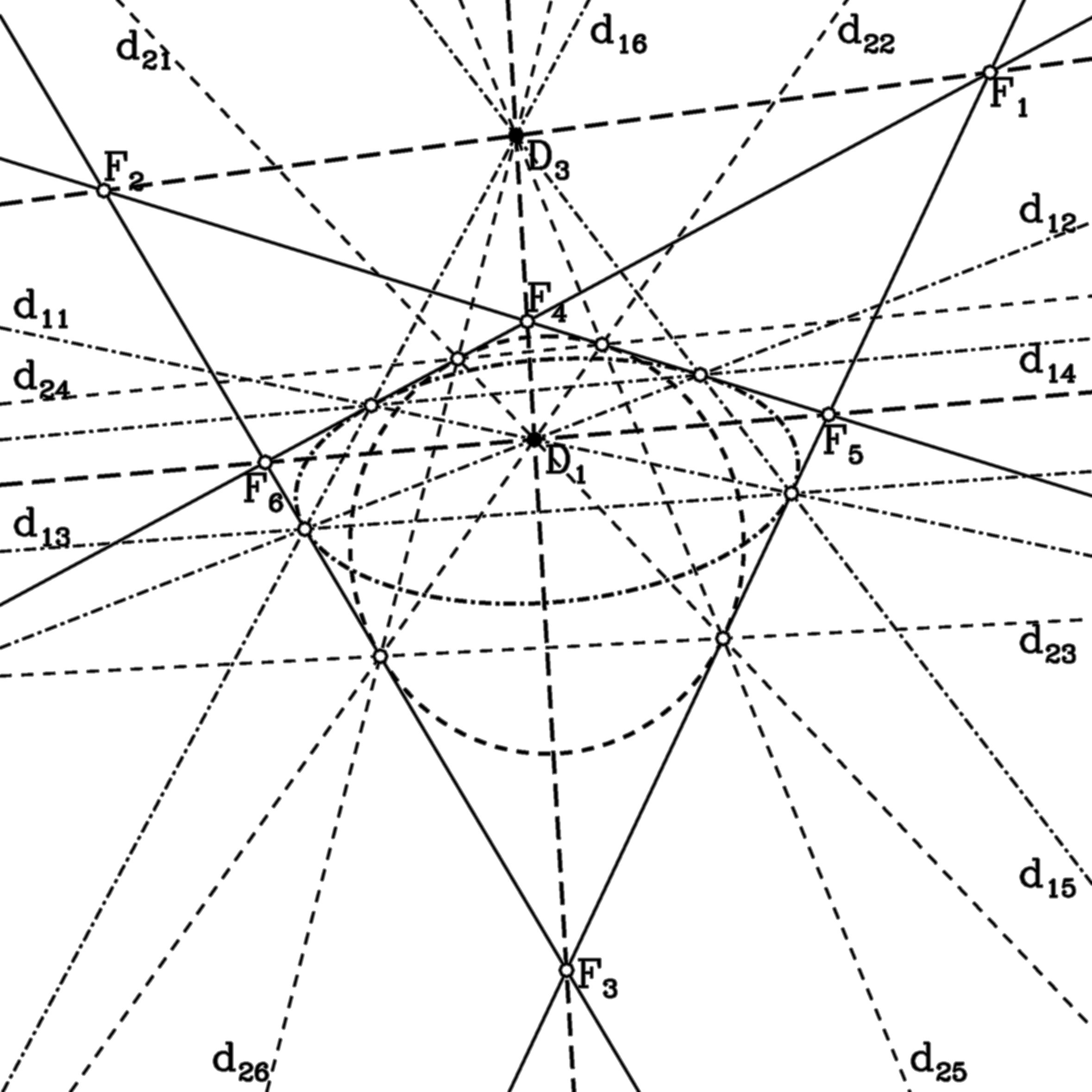## Directrices of conics

Two conics have four common tangents and three pairs of foci. The directrices to these foci are the 12 polars with respect to the two conics. They meet in groups of four in the diagonal points of the quadrilateral of common tangents.

For instance, in D1 = (F3xF4)x(F5xF6) meet the directrices of F1 and F2 with respect to the two conics.

The triangle of the diagonal points is self-polar with respect to both the conics. It coincides with the diagonal triangle of the quadrangle of the four intersection points.